Function Repository Resource:

# ToInversionVector

Get the inversion vector of a permutation written as a list

Contributed by: Wolfram Staff
 ResourceFunction["ToInversionVector"][p] gives the inversion vector of the permutation list p.

## Details and Options

The inversion vector of a permutation of length n lists the number of times k is preceded by an entry greater than k, where k runs from 1 to n.

## Examples

### Basic Examples (2)

This permutation has 3 numbers greater than 1 before 1, 2 numbers greater than 2 before 2, and so on:

 In:=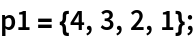Therefore, this is its inversion vector:

 In:=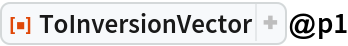Out=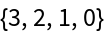Here is another example:

 In:=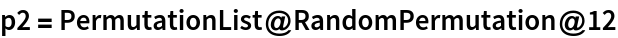Out=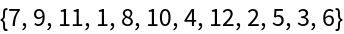In:=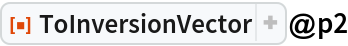Out=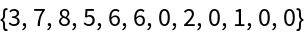### Scope (3)

Here are all six permutation of {1,2,3}:

 In:=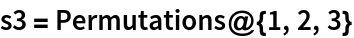Out=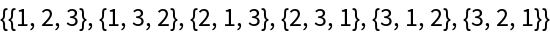Here are the corresponding inversion vectors:

 In:=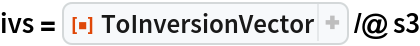Out=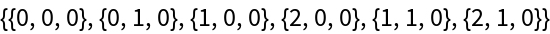In the factorial base, ivs represents the numbers from 0 to 3!-1:

 In:=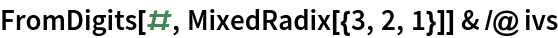Out=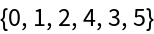George Beck

## Version History

• 1.0.0 – 31 May 2019

## Author Notes

The Combinatorica` function ToInversionVector did not include the final zero.